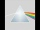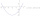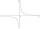# Mysterious number

The magician thinks the number: "The mysterious number is first divided by minus five, dividing the result by three, multiplying the number by ten, and dividing the resulting number by minus four. This gives result 5.
Can you reveal the mysterious number?

x =  30

### Step-by-step explanation:

x/(-5) / 3 • 10/(-4) = 5

0.5x = 15

x = 30

Our simple equation calculator calculates it.Did you find an error or inaccuracy? Feel free to write us. Thank you!Tips to related online calculators
Do you have a linear equation or system of equations and looking for its solution? Or do you have a quadratic equation?

## Related math problems and questions:Please determine the solvability conditions of the equation, solve the equation and perform the test: x divided by x squared minus 2x plus1 the whole minus x + 3 divided by x squared minus 1 this is equal to 0: x/(x2-2x+1) - (x+3)/( x2-1) = 0
• MistakeNicol mistake when calculate in school. Instead of add number 20 subtract it. What is the difference between the result and the right result?
• Result and remainderAfter dividing the unknown number by the number 23, the quotient 11 and the remainder 4 are formed. Find an unknown number.
• NumberCalculate the integer number which, divided by 34 gives 10 and the rest 25.
• Six times6 times a number has 2 subtracted from it. The result it thrilled . the answer is the same if you add 6 to twice the number and multiply the result by 2
• Unknown numberSamuel wrote unknown number. Then he had add 200000 to the number and the result multiply by three. When it calculated he was surprised, because the result would have received anyway, if write digit to the end of original number. Find unknown number.
• Unknown number 5Daniel thinks an integer. When he change this number at a ratio of 2:5, he got the number 2.8. Determine what number think Daniel.
• Unknown numberI think the number. I'll reduce it to its one-third. The result is then increased by one-third, and I get the number 12.
• SixteenSixteen minus the quotient of 12 and what number is thirteen?
• DivisibilityIs the number 761082 exactly divisible by 9? (the result is the integer and/or remainder is zero)
• Diofant equationIn the set of integers (Z) solve the equation: 212x +316y =0 Write result with integer parameter t in Z (parameter t = ...-2,-1,0,1,2,3... if equation has infinitely many solutions)
• Taken away from number18 is taken away from 8 times of a number is 30. Find the number.
• The number 4Two-thirds of a number is negative six. Find the number.
• EquationSolve the equation: 1/2-2/8 = 1/10; Write the result as a decimal number.
• Original numberJoe divided a number by 2 1/4 to get 2/3. What was the original number?
• Unknown number 5I think of an unknown number. If we enlarge it five times then subtract 3 and the result decreases by 75% we get one greater than the number. What number am I thinking of?
• Sign of a expressionWhat is the sign of expression: minus 18 start fraction 9 divided by 17 end fraction plus left parenthesis minus 18 start fraction 9 divided by 17 end fraction right parenthesis?